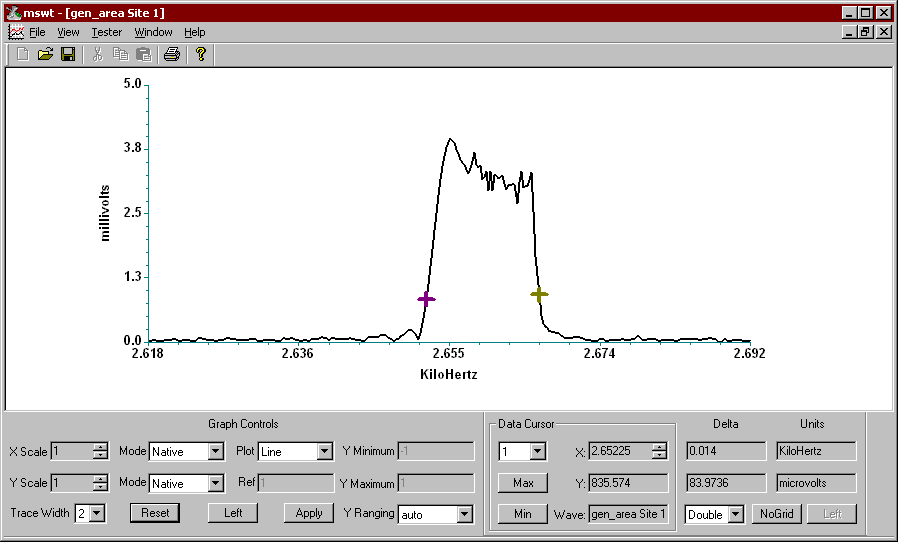Below you see the spectrum of the Sonalert on the train as it "streaks" past the microphone that is recording its passage on the track. Lower frequencies are on the left, higher frequencies are on the right. You can see a bit of a spread spectrum, that's due to the Doppler shift as the train goes past the microphone. The actual frequency of the Sonalert is right in the middle, but we don't get a spike there because the train was always in motion during this capture. So, as the train approaches the microphone, the frequency is shifted higher, that causes the signal on the right side of center, and as the train begins to recede from the microphone it causes the signal on the left side of center.

The formula uses the Speed of Sound (SOS) as a constant to calculate the speed of the train. The simple version of the formula is speed = speed of sound * delta_wavelength/wavelength, but we have to do the algebra because we don't get wavelength, we get the answers in frequency.Measuring speed by Doppler effect Speed = SOS*(Δλ/λ)SOS = Speed of Sound = 771 MPH